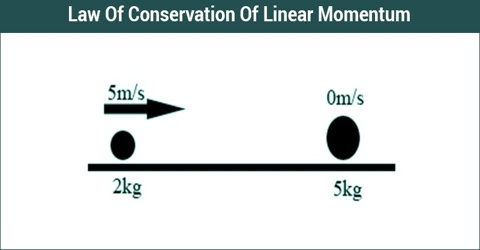Physics

# Conservation Principle of Linear MomentumConservation principle of linear momentum:

Momentum is the motion contained in a body. Quantity of motion possessed by a body depends upon both of its mass and velocity. So the product of mass and velocity is the measure of the momentum,

P = mv

It is also called linear momentum. It is a vector quantity whose direction is in the direction of the velocity. Its unit is kg m/s is SI-units and dimension, [M L T-1].

The principle of conservation of linear momentum states that,

“If no external forces act on the system of two colliding objects, then the vector sum of the linear momentum of each body remains constant and is not affected by their mutual interaction”.

Linear momentum of a system has constant magnitude and direction if the system is subjected to no external force. Also called law of conservation of linear momentum. If no external force is active on bodies or if net applied external force is zero, then total linear momentum of those bodies will remain constant, or there will not be any change of momentum. Look at the following examples and you will be able to explain how conservation principle of linear momentum is effective there.

Example 1: When a bullet is fired from cannon, it goes ahead with tremendous velocity. Before firing both the cannon and the bullet were at rest, so momentum was zero. But after firing the cannon the bullet acquires a momentum. The cannon acquire equal but oppositely directed momentum. Because of it the cannon recoils back [Figure].Example 2: When a passenger jumps from a boat to the bank, the boat pushes back. Before jump the boat and the passenger were at rest, so their total momentum was zero. As the passenger jumps, he becomes mobile and acquires momentum. According to the principle at conservation of momentum, total momentum is zero. So, there occurs equal and oppositely directed momentum in the boat. Hence the boat bring mobile pushes back (Fig)